# Precalculus : Polynomial Functions

## Example Questions

### Example Question #6 : Express A Polynomial As A Product Of Linear Factors

Factorize the following expression completely to its linear factors: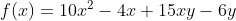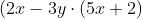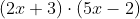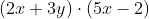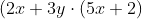Explanation:

Use the grouping method to factorize common terms: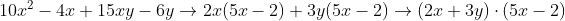### Example Question #7 : Express A Polynomial As A Product Of Linear Factors

Factorize the following expression completely to its linear factors: f(x)=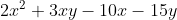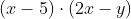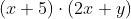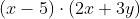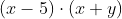Explanation:

Use grouping method to factorize common terms: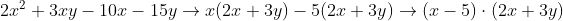### Example Question #8 : Express A Polynomial As A Product Of Linear Factors

Express the polynomial as a product of linear factors: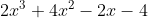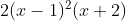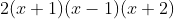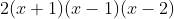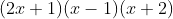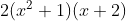Explanation:

First pull out the common factor of 2, and then factorize:### Example Question #1 : Express A Polynomial As A Product Of Linear Factors

Find the real zeros of the equation using factorization: f(x)=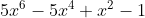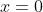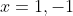There are no real zeros.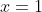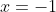Explanation:

Use grouping to factorize the common terms: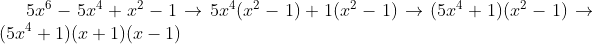### Example Question #10 : Express A Polynomial As A Product Of Linear Factors

Express the following polynomial as a product of linear factors:Explanation:

First, pull out the common factor of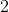, and then factorize: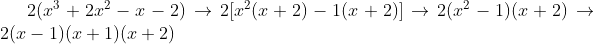### Example Question #11 : Fundamental Theorem Of Algebra

Factorize the following expression completely to its linear factors: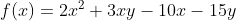Explanation:

Use the grouping method to factorize common terms: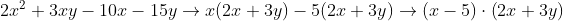### Example Question #12 : Fundamental Theorem Of Algebra

Factorize the following polynomial expression completely to its linear factors:Explanation:

Use the grouping method to factorize common terms: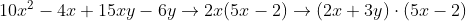### Example Question #1 : Find Complex Zeros Of A Polynomial Using The Fundamental Theorem Of Algebra

What are the roots of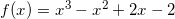including complex roots, if they exist?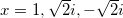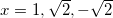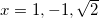Explanation:

One of the roots isbecause if we plug in 1, we get 0. We can factor the polynomial as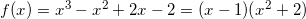So now we solve the roots of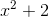.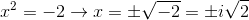The root will not be real.

The roots of this polynomial are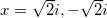.

So, the roots are### Example Question #2 : Find Complex Zeros Of A Polynomial Using The Fundamental Theorem Of Algebra

The polynomial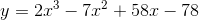has a real zero at 1.5. Find the other two zeros.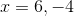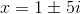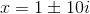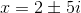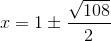Explanation:

If this polynomial has a real zero at 1.5, that means that the polynomial has a factor that when set equal to zero has a solution of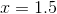. We can figure out what this is this way: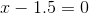multiply both sides by 2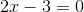is the factor

Now that we have one factor, we can divide to find the other two solutions: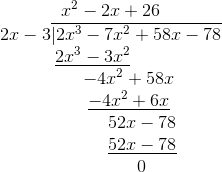To finish solving, we can use the quadratic formula with the resulting quadratic,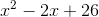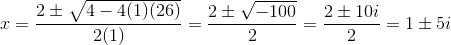### Example Question #1 : Find Complex Zeros Of A Polynomial Using The Fundamental Theorem Of Algebra

If the real zero of the polynomial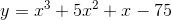is 3, what are the complex zeros?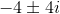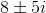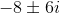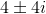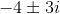Explanation:

We know that the real zero of this polynomial is 3, so one of the factors must be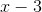. To find the other factors, we can divide the original polynomial by, either by long division or synthetic division: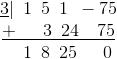This gives us a second factor of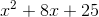which we can solve using the quadratic formula: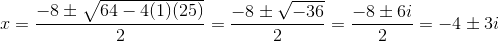### All Precalculus Resources